# Tarjan’s off-line lowest common ancestors algorithm

• Difficulty Level : Expert
• Last Updated : 02 Mar, 2022

Prerequisite : LCA basics, Disjoint Set Union by Rank and Path Compression
We are given a tree(can be extended to a DAG) and we have many queries of form LCA(u, v), i.e., find LCA of nodes ‘u’ and ‘v’.
We can perform those queries in O(N + QlogN) time using RMQ, where O(N) time for pre-processing and O(log N) for answering the queries, where
N = number of nodes and
Q = number of queries to be answered.
Can we do better than this? Can we do in linear(almost) time? Yes.
The article presents an offline algorithm which performs those queries in approximately O(N + Q) time. Although, this is not exactly linear, as there is an Inverse Ackermann function involved in the time complexity analysis. For more details on Inverse Ackermann function see this. Just as a summary, we can say that the Inverse Ackermann Function remains less than 4, for any value of input size that can be written in physical inverse. Thus, we consider this as almost linear.
We consider the input tree as shown below. We will Pre-Process the tree and fill two arrays- child[] and sibling[] according to the below explanation-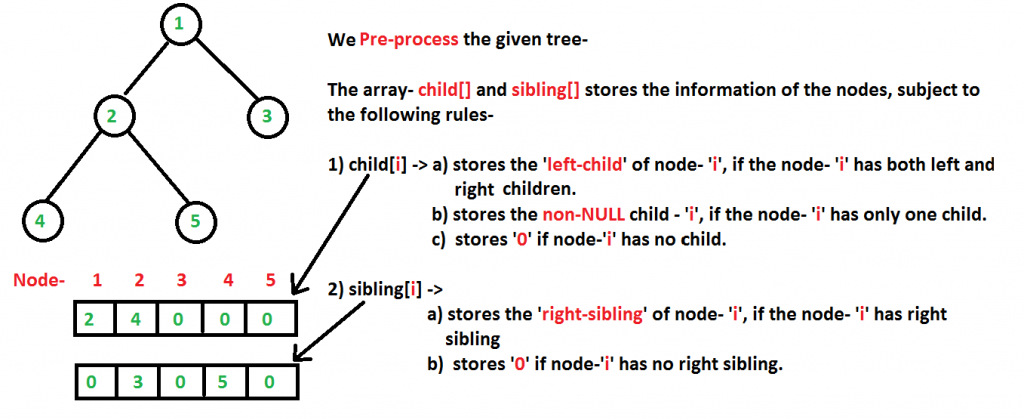Let we want to process these queries- LCA(5,4), LCA(1,3), LCA(2,3)
Now, after pre-processing, we perform a LCA walk starting from the root of the tree(here- node ‘1’). But prior to the LCA walk, we colour all the nodes with WHITE. During the whole LCA walk, we use three disjoint set union functions- makeSet(), findSet(), unionSet().
These functions use the technique of union by rank and path compression to improve the running time. During the LCA walk, our queries gets processed and outputted (in a random order). After the LCA walk of the whole tree, all the nodes gets coloured BLACK.
Tarjan Offline LCA Algorithm steps from CLRS, Section-21-3, Pg 584, 2nd /3rd edition.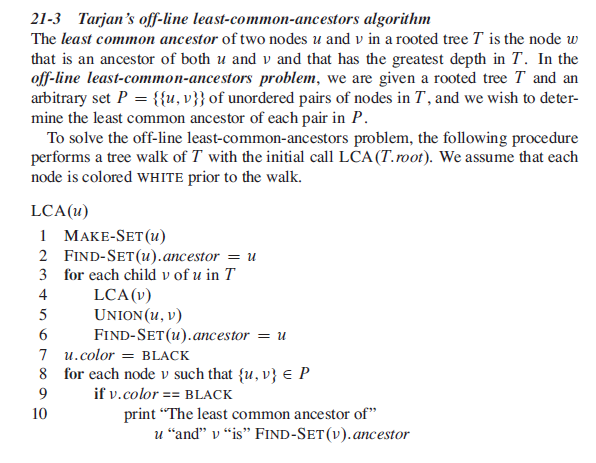Note- The queries may not be processed in the original order. We can easily modify the process and sort them according to the input order.
The below pictures clearly depict all the steps happening. The red arrow shows the direction of travel of our recursive function LCA()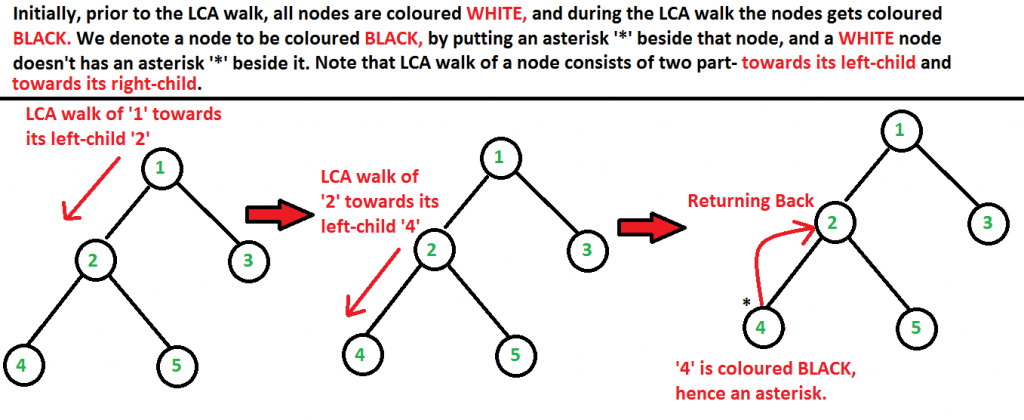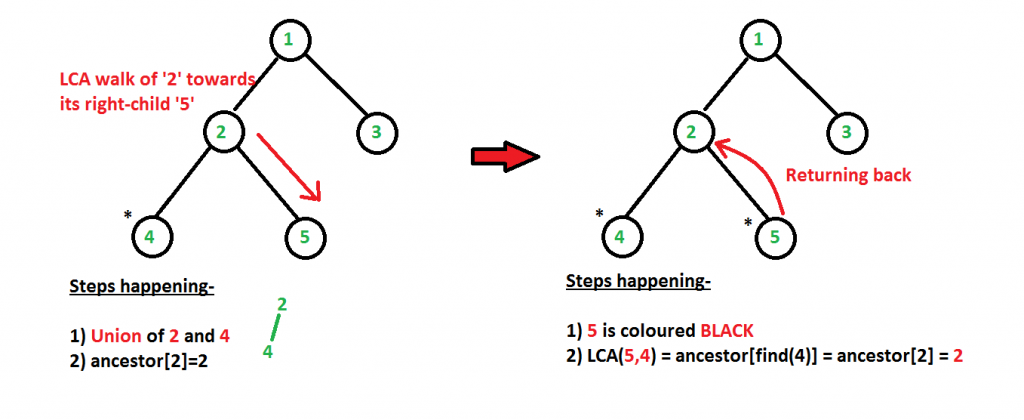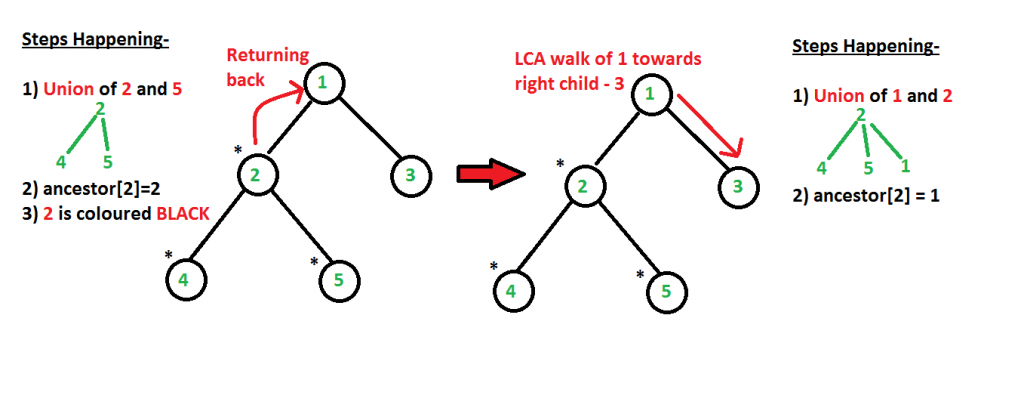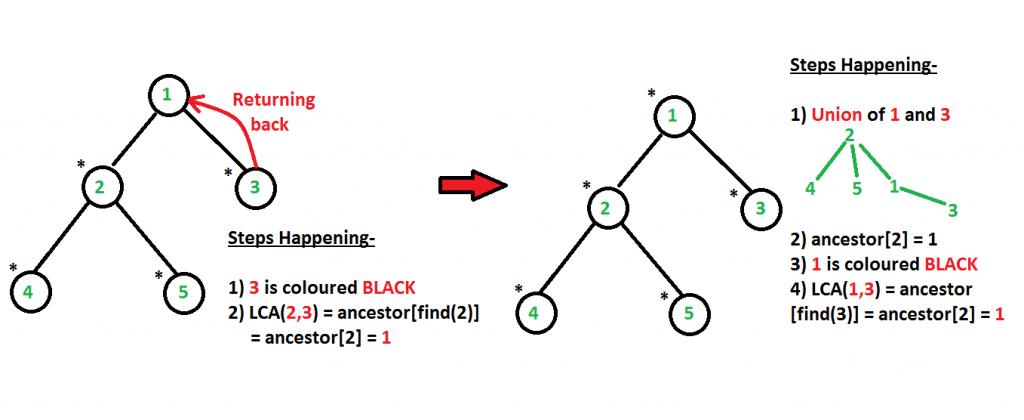As, we can clearly see from the above pictures, the queries are processed in the following order, LCA(5,4), LCA(2,3), LCA(1,3) which is not in the same order as the input(LCA(5,4), LCA(1,3), LCA(2,3)).

Below is the implementation of the above approach:

## C++

 `// A C++ Program to implement Tarjan Offline LCA Algorithm` `#include `   `#define V 5       // number of nodes in input tree` `#define WHITE 1      // COLOUR 'WHITE' is assigned value 1` `#define BLACK 2   // COLOUR 'BLACK' is assigned value 2`   `/* A binary tree node has data, pointer to left child` `   ``and a pointer to right child */` `struct` `Node` `{` `    ``int` `data;` `    ``Node* left, *right;` `};`   `/*` ` ``subset[i].parent-->Holds the parent of node-'i'` ` ``subset[i].rank-->Holds the rank of node-'i'` ` ``subset[i].ancestor-->Holds the LCA queries answers` ` ``subset[i].child-->Holds one of the child of node-'i'` `                     ``if present, else -'0'` ` ``subset[i].sibling-->Holds the right-sibling of node-'i'` `                     ``if present, else -'0'` ` ``subset[i].color-->Holds the colour of node-'i'` `*/` `struct` `subset` `{` `    ``int` `parent, rank, ancestor, child, sibling, color;` `};`   `// Structure to represent a query` `// A query consists of (L,R) and we will process the` `// queries offline a/c to Tarjan's offline LCA algorithm` `struct` `Query` `{` `    ``int` `L, R;` `};`   `/* Helper function that allocates a new node with the` `   ``given data and NULL left and right pointers. */` `Node* newNode(``int` `data)` `{` `    ``Node* node = ``new` `Node;` `    ``node->data = data;` `    ``node->left = node->right = NULL;` `    ``return``(node);` `}`   `//A utility function to make set` `void` `makeSet(``struct` `subset subsets[], ``int` `i)` `{` `    ``if` `(i < 1 || i > V)` `        ``return``;`   `    ``subsets[i].color = WHITE;` `    ``subsets[i].parent = i;` `    ``subsets[i].rank = 0;`   `    ``return``;` `}`   `// A utility function to find set of an element i` `// (uses path compression technique)` `int` `findSet(``struct` `subset subsets[], ``int` `i)` `{` `    ``// find root and make root as parent of i (path compression)` `    ``if` `(subsets[i].parent != i)` `        ``subsets[i].parent = findSet (subsets, subsets[i].parent);`   `    ``return` `subsets[i].parent;` `}`   `// A function that does union of two sets of x and y` `// (uses union by rank)` `void` `unionSet(``struct` `subset subsets[], ``int` `x, ``int` `y)` `{` `    ``int` `xroot = findSet (subsets, x);` `    ``int` `yroot = findSet (subsets, y);`   `    ``// Attach smaller rank tree under root of high rank tree` `    ``// (Union by Rank)` `    ``if` `(subsets[xroot].rank < subsets[yroot].rank)` `        ``subsets[xroot].parent = yroot;` `    ``else` `if` `(subsets[xroot].rank > subsets[yroot].rank)` `        ``subsets[yroot].parent = xroot;`   `    ``// If ranks are same, then make one as root and increment` `    ``// its rank by one` `    ``else` `    ``{` `        ``subsets[yroot].parent = xroot;` `        ``(subsets[xroot].rank)++;` `    ``}` `}`   `// The main function that prints LCAs. u is root's data.` `// m is size of q[]` `void` `lcaWalk(``int` `u, ``struct` `Query q[], ``int` `m,` `             ``struct` `subset subsets[])` `{` `    ``// Make Sets` `    ``makeSet(subsets, u);`   `    ``// Initially, each node's ancestor is the node` `    ``// itself.` `    ``subsets[findSet(subsets, u)].ancestor = u;`   `    ``int` `child = subsets[u].child;`   `    ``// This while loop doesn't run for more than 2 times` `    ``// as there can be at max. two children of a node` `    ``while` `(child != 0)` `    ``{` `        ``lcaWalk(child, q, m, subsets);` `        ``unionSet (subsets, u, child);` `        ``subsets[findSet(subsets, u)].ancestor = u;` `        ``child = subsets[child].sibling;` `    ``}`   `    ``subsets[u].color = BLACK;`   `    ``for` `(``int` `i = 0; i < m; i++)` `    ``{` `        ``if` `(q[i].L == u)` `        ``{` `            ``if` `(subsets[q[i].R].color == BLACK)` `            ``{` `                ``printf``(``"LCA(%d %d) -> %d\n"``,` `                  ``q[i].L,` `                  ``q[i].R,` `                  ``subsets[findSet(subsets,q[i].R)].ancestor);` `            ``}` `        ``}` `        ``else` `if` `(q[i].R == u)` `        ``{` `            ``if` `(subsets[q[i].L].color == BLACK)` `            ``{` `                ``printf``(``"LCA(%d %d) -> %d\n"``,` `                  ``q[i].L,` `                  ``q[i].R,` `                  ``subsets[findSet(subsets,q[i].L)].ancestor);` `            ``}` `        ``}` `    ``}`   `    ``return``;` `}`   `// This is basically an inorder traversal and` `// we preprocess the arrays-> child[]` `// and sibling[] in "struct subset" with` `// the tree structure using this function.` `void` `preprocess(Node * node, ``struct` `subset subsets[])` `{` `    ``if` `(node == NULL)` `        ``return``;`   `    ``// Recur on left child` `    ``preprocess(node->left, subsets);`   `    ``if` `(node->left != NULL&&node->right != NULL)` `    ``{` `        ``/* Note that the below two lines can also be this-` `        ``subsets[node->data].child = node->right->data;` `        ``subsets[node->right->data].sibling =` `                                         ``node->left->data;`   `        ``This is because if both left and right children of` `        ``node-'i' are present then we can store any of them` `        ``in subsets[i].child and correspondingly its sibling*/` `        ``subsets[node->data].child = node->left->data;` `        ``subsets[node->left->data].sibling =` `            ``node->right->data;`   `    ``}` `    ``else` `if` `((node->left != NULL && node->right == NULL)` `             ``|| (node->left == NULL && node->right != NULL))` `    ``{` `        ``if``(node->left != NULL && node->right == NULL)` `            ``subsets[node->data].child = node->left->data;` `        ``else` `            ``subsets[node->data].child = node->right->data;` `    ``}`   `    ``//Recur on right child` `    ``preprocess (node->right, subsets);` `}`   `// A function to initialise prior to pre-processing and` `// LCA walk` `void` `initialise(``struct` `subset subsets[])` `{` `    ``// Initialising the structure with 0's` `    ``memset``(subsets, 0, (V+1) * ``sizeof``(``struct` `subset));`   `    ``// We colour all nodes WHITE before LCA Walk.` `    ``for` `(``int` `i=1; i<=V; i++)` `        ``subsets[i].color=WHITE;`   `    ``return``;` `}`   `// Prints LCAs for given queries q[0..m-1] in a tree` `// with given root` `void` `printLCAs(Node *root, Query q[], ``int` `m)` `{` `    ``// Allocate memory for V subsets and nodes` `    ``struct` `subset * subsets = ``new` `subset[V+1];`   `    ``// Creates subsets and colors them WHITE` `    ``initialise(subsets);`   `    ``// Preprocess the tree` `    ``preprocess(root, subsets);`   `    ``// Perform a tree walk to process the LCA queries` `    ``// offline` `    ``lcaWalk(root->data , q, m, subsets);` `}`   `// Driver program to test above functions` `int` `main()` `{` `    ``/*` `     ``We construct a binary tree :-` `            ``1` `          ``/  \` `         ``2    3` `       ``/  \` `      ``4    5           */`   `    ``Node *root = newNode(1);` `    ``root->left        = newNode(2);` `    ``root->right       = newNode(3);` `    ``root->left->left  = newNode(4);` `    ``root->left->right = newNode(5);`   `    ``// LCA Queries to answer` `    ``Query q[] = {{5, 4}, {1, 3}, {2, 3}};` `    ``int` `m = ``sizeof``(q)/``sizeof``(q);`   `    ``printLCAs(root, q, m);`   `    ``return` `0;` `}`

## Java

 `// A Java Program to implement Tarjan Offline LCA Algorithm` `import` `java.util.Arrays;` `class` `GFG` `{` `static` `final` `int` `V = ``5``;       ``// number of nodes in input tree` `static` `final` `int` `WHITE = ``1``;      ``// COLOUR 'WHITE' is assigned value 1` `static` `final` `int` `BLACK = ``2``;   ``// COLOUR 'BLACK' is assigned value 2`   `/* A binary tree node has data, pointer to left child` `   ``and a pointer to right child */` `static` `class` `Node` `{` `    ``int` `data;` `    ``Node left, right;` `};`   `/*` ` ``subset[i].parent-.Holds the parent of node-'i'` ` ``subset[i].rank-.Holds the rank of node-'i'` ` ``subset[i].ancestor-.Holds the LCA queries answers` ` ``subset[i].child-.Holds one of the child of node-'i'` `                     ``if present, else -'0'` ` ``subset[i].sibling-.Holds the right-sibling of node-'i'` `                     ``if present, else -'0'` ` ``subset[i].color-.Holds the colour of node-'i'` `*/` `static` `class` `subset` `{` `    ``int` `parent;` `    ``int` `rank;` `    ``int` `ancestor;` `    ``int` `child;` `    ``int` `sibling;` `    ``int` `color;` `};`   `// Structure to represent a query` `// A query consists of (L,R) and we will process the` `// queries offline a/c to Tarjan's offline LCA algorithm` `static` `class` `Query` `{` `    ``int` `L, R;` `    ``Query(``int` `L, ``int` `R)` `    ``{` `        ``this``.L = L;` `        ``this``.R = R;` `    ``}` `};`   `/* Helper function that allocates a new node with the` `   ``given data and null left and right pointers. */` `static` `Node newNode(``int` `data)` `{` `    ``Node node = ``new` `Node();` `    ``node.data = data;` `    ``node.left = node.right = ``null``;` `    ``return``(node);` `}`   `// A utility function to make set` `static` `void` `makeSet(subset subsets[], ``int` `i)` `{` `    ``if` `(i < ``1` `|| i > V)` `        ``return``;` `    ``subsets[i].color = WHITE;` `    ``subsets[i].parent = i;` `    ``subsets[i].rank = ``0``;` `    ``return``;` `}`   `// A utility function to find set of an element i` `// (uses path compression technique)` `static` `int` `findSet(subset subsets[], ``int` `i)` `{` `    ``// find root and make root as parent of i (path compression)` `    ``if` `(subsets[i].parent != i)` `        ``subsets[i].parent = findSet (subsets, subsets[i].parent);`   `    ``return` `subsets[i].parent;` `}`   `// A function that does union of two sets of x and y` `// (uses union by rank)` `static` `void` `unionSet(subset subsets[], ``int` `x, ``int` `y)` `{` `    ``int` `xroot = findSet (subsets, x);` `    ``int` `yroot = findSet (subsets, y);`   `    ``// Attach smaller rank tree under root of high rank tree` `    ``// (Union by Rank)` `    ``if` `(subsets[xroot].rank < subsets[yroot].rank)` `        ``subsets[xroot].parent = yroot;` `    ``else` `if` `(subsets[xroot].rank > subsets[yroot].rank)` `        ``subsets[yroot].parent = xroot;`   `    ``// If ranks are same, then make one as root and increment` `    ``// its rank by one` `    ``else` `    ``{` `        ``subsets[yroot].parent = xroot;` `        ``(subsets[xroot].rank)++;` `    ``}` `}`   `// The main function that prints LCAs. u is root's data.` `// m is size of q[]` `static` `void` `lcaWalk(``int` `u, Query q[], ``int` `m,` `             ``subset subsets[])` `{` `  `  `    ``// Make Sets` `    ``makeSet(subsets, u);`   `    ``// Initially, each node's ancestor is the node` `    ``// itself.` `    ``subsets[findSet(subsets, u)].ancestor = u;` `    ``int` `child = subsets[u].child;`   `    ``// This while loop doesn't run for more than 2 times` `    ``// as there can be at max. two children of a node` `    ``while` `(child != ``0``)` `    ``{` `        ``lcaWalk(child, q, m, subsets);` `        ``unionSet (subsets, u, child);` `        ``subsets[findSet(subsets, u)].ancestor = u;` `        ``child = subsets[child].sibling;` `    ``}`   `    ``subsets[u].color = BLACK;` `    ``for` `(``int` `i = ``0``; i < m; i++)` `    ``{` `        ``if` `(q[i].L == u)` `        ``{` `            ``if` `(subsets[q[i].R].color == BLACK)` `            ``{` `                ``System.out.printf(``"LCA(%d %d)->%d\n"``,` `                  ``q[i].L,` `                  ``q[i].R,` `                  ``subsets[findSet(subsets,q[i].R)].ancestor);` `            ``}` `        ``}` `        ``else` `if` `(q[i].R == u)` `        ``{` `            ``if` `(subsets[q[i].L].color == BLACK)` `            ``{` `                ``System.out.printf(``"LCA(%d %d)->%d\n"``,` `                  ``q[i].L,` `                  ``q[i].R,` `                  ``subsets[findSet(subsets,q[i].L)].ancestor);` `            ``}` `        ``}` `    ``}` `    ``return``;` `}`   `// This is basically an inorder traversal and` `// we preprocess the arrays. child[]` `// and sibling[] in "subset" with` `// the tree structure using this function.` `static` `void` `preprocess(Node  node, subset subsets[])` `{` `    ``if` `(node == ``null``)` `        ``return``;`   `    ``// Recur on left child` `    ``preprocess(node.left, subsets);`   `    ``if` `(node.left != ``null` `&& node.right != ``null``)` `    ``{` `      `  `        ``/* Note that the below two lines can also be this-` `        ``subsets[node.data].child = node.right.data;` `        ``subsets[node.right.data].sibling =` `                                         ``node.left.data;`   `        ``This is because if both left and right children of` `        ``node-'i' are present then we can store any of them` `        ``in subsets[i].child and correspondingly its sibling*/` `        ``subsets[node.data].child = node.left.data;` `        ``subsets[node.left.data].sibling =` `            ``node.right.data;`   `    ``}` `    ``else` `if` `((node.left != ``null` `&& node.right == ``null``)` `             ``|| (node.left == ``null` `&& node.right != ``null``))` `    ``{` `        ``if``(node.left != ``null` `&& node.right == ``null``)` `            ``subsets[node.data].child = node.left.data;` `        ``else` `            ``subsets[node.data].child = node.right.data;` `    ``}`   `    ``// Recur on right child` `    ``preprocess (node.right, subsets);` `}`   `// A function to initialise prior to pre-processing and` `// LCA walk` `static` `void` `initialise(subset subsets[])` `{` `  `  `    ``// We colour all nodes WHITE before LCA Walk.` `    ``for` `(``int` `i = ``1``; i < subsets.length; i++)` `    ``{` `        ``subsets[i] = ``new` `subset();` `        ``subsets[i].color = WHITE;` `    ``}` `    ``return``;` `}`   `// Prints LCAs for given queries q[0..m-1] in a tree` `// with given root` `static` `void` `printLCAs(Node root, Query q[], ``int` `m)` `{` `  `  `    ``// Allocate memory for V subsets and nodes` `    ``subset  []subsets = ``new` `subset[V + ``1``];`   `    ``// Creates subsets and colors them WHITE` `    ``initialise(subsets);`   `    ``// Preprocess the tree` `    ``preprocess(root, subsets);`   `    ``// Perform a tree walk to process the LCA queries` `    ``// offline` `    ``lcaWalk(root.data , q, m, subsets);` `}`   `// Driver code` `public` `static` `void` `main(String[] args)` `{` `    ``/*` `     ``We construct a binary tree :-` `            ``1` `          ``/  \` `         ``2    3` `       ``/  \` `      ``4    5           */`   `    ``Node root = newNode(``1``);` `    ``root.left = newNode(``2``);` `    ``root.right = newNode(``3``);` `    ``root.left.left = newNode(``4``);` `    ``root.left.right = newNode(``5``);`   `    ``// LCA Queries to answer` `    ``Query q[] =  ``new` `Query[``3``];` `    ``q[``0``] = ``new` `Query(``5``, ``4``);` `    ``q[``1``] = ``new` `Query(``1``, ``3``);` `    ``q[``2``] = ``new` `Query(``2``, ``3``);` `    ``int` `m = q.length;` `    ``printLCAs(root, q, m);` `}` `}`   `// This code is contributed by gauravrajput1 `

## Python3

 `# A Python3 program to implement Tarjan` `# Offline LCA Algorithm`   `# Number of nodes in input tree` `V ``=` `5`   `# COLOUR 'WHITE' is assigned value 1` `WHITE ``=` `1`   `# COLOUR 'BLACK' is assigned value 2` `BLACK ``=` `2`    `# A binary tree node has data, pointer ` `# to left child and a pointer to right child` `class` `Node:` `    `  `    ``def` `__init__(``self``):`   `        ``self``.data ``=` `0` `        ``self``.left ``=` `None` `        ``self``.right ``=` `None`   `'''` ` ``subset[i].parent-.Holds the parent of node-'i'` ` ``subset[i].rank-.Holds the rank of node-'i'` ` ``subset[i].ancestor-.Holds the LCA queries answers` ` ``subset[i].child-.Holds one of the child of node-'i'` `                     ``if present, else -'0'` ` ``subset[i].sibling-.Holds the right-sibling of node-'i'` `                     ``if present, else -'0'` ` ``subset[i].color-.Holds the colour of node-'i'` `'''` `class` `subset:` `    `  `    ``def` `__init__(``self``):`   `        ``self``.parent ``=` `0` `        ``self``.rank ``=` `0` `        ``self``.ancestor ``=` `0` `        ``self``.child ``=` `0` `        ``self``.sibling ``=` `0` `        ``self``.color ``=` `0`   `# Structure to represent a query` `# A query consists of (L,R) and we` `# will process the queries offline ` `# a/c to Tarjan's offline LCA algorithm` `class` `Query:` `    `  `    ``def` `__init__(``self``, L, R):`   `        ``self``.L ``=` `L` `        ``self``.R ``=` `R`   `# Helper function that allocates a new node` `# with the given data and None left and ` `# right pointers. ` `def` `newNode(data):`   `    ``node ``=` `Node()` `    ``node.data ``=` `data` `    ``node.left ``=` `node.right ``=` `None` `    ``return` `(node)`   `# A utility function to make set` `def` `makeSet(subsets, i):`   `    ``if` `(i < ``1` `or` `i > V):` `        ``return`   `    ``subsets[i].color ``=` `WHITE` `    ``subsets[i].parent ``=` `i` `    ``subsets[i].rank ``=` `0`   `    ``return`   `# A utility function to find set of an element i` `# (uses path compression technique)` `def` `findSet(subsets, i):`   `    ``# Find root and make root as parent` `    ``# of i (path compression)` `    ``if` `(subsets[i].parent !``=` `i):` `        ``subsets[i].parent ``=` `findSet(subsets, ` `                                    ``subsets[i].parent)`   `    ``return` `subsets[i].parent`   `# A function that does union of two sets ` `# of x and y (uses union by rank)` `def` `unionSet(subsets, x, y):`   `    ``xroot ``=` `findSet(subsets, x)` `    ``yroot ``=` `findSet(subsets, y)`   `    ``# Attach smaller rank tree under root of ` `    ``# high rank tree (Union by Rank)` `    ``if` `(subsets[xroot].rank < subsets[yroot].rank):` `        ``subsets[xroot].parent ``=` `yroot` `    ``else` `if` `(subsets[xroot].rank > subsets[yroot].rank):` `        ``subsets[yroot].parent ``=` `xroot`   `    ``# If ranks are same, then make one as root` `    ``# and increment its rank by one` `    ``else``:` `        ``subsets[yroot].parent ``=` `xroot` `        ``(subsets[xroot].rank) ``+``=` `1`   `# The main function that prints LCAs. u is` `# root's data. m is size of q[]` `def` `lcaWalk(u, q, m, subsets):`   `    ``# Make Sets` `    ``makeSet(subsets, u)`   `    ``# Initially, each node's ancestor is the node` `    ``# itself.` `    ``subsets[findSet(subsets, u)].ancestor ``=` `u`   `    ``child ``=` `subsets[u].child`   `    ``# This while loop doesn't run for more than 2 times` `    ``# as there can be at max. two children of a node` `    ``while` `(child !``=` `0``):` `        ``lcaWalk(child, q, m, subsets)` `        ``unionSet(subsets, u, child)` `        ``subsets[findSet(subsets, u)].ancestor ``=` `u` `        ``child ``=` `subsets[child].sibling`   `    ``subsets[u].color ``=` `BLACK`   `    ``for` `i ``in` `range``(m):` `        ``if` `(q[i].L ``=``=` `u):` `            ``if` `(subsets[q[i].R].color ``=``=` `BLACK):`   `                ``print``(``"LCA(%d %d) -> %d"` `%` `(q[i].L, q[i].R, ` `                   ``subsets[findSet(subsets, q[i].R)].ancestor))`   `        ``else` `if` `(q[i].R ``=``=` `u):` `            ``if` `(subsets[q[i].L].color ``=``=` `BLACK):`   `                ``print``(``"LCA(%d %d) -> %d"` `%` `(q[i].L, q[i].R,` `                   ``subsets[findSet(subsets, q[i].L)].ancestor))`   `    ``return`   `# This is basically an inorder traversal and` `# we preprocess the arrays. child[]` `# and sibling[] in "struct subset" with` `# the tree structure using this function.` `def` `preprocess(node, subsets):`   `    ``if` `(node ``=``=` `None``):` `        ``return`   `    ``# Recur on left child` `    ``preprocess(node.left, subsets)`   `    ``if` `(node.left !``=` `None` `and` `node.right !``=` `None``):` `        `  `        ``''' Note that the below two lines can also be this-` `        ``subsets[node.data].child = node.right.data;` `        ``subsets[node.right.data].sibling =` `                                         ``node.left.data;`   `        ``This is because if both left and right children of` `        ``node-'i' are present then we can store any of them` `        ``in subsets[i].child and correspondingly its sibling'''` `        ``subsets[node.data].child ``=` `node.left.data` `        ``subsets[node.left.data].sibling ``=` `node.right.data`   `    ``else` `if` `((node.left !``=` `None` `and` `node.right ``=``=` `None``)` `          ``or` `(node.left ``=``=` `None` `and` `node.right !``=` `None``)):`   `        ``if` `(node.left !``=` `None` `and` `node.right ``=``=` `None``):` `            ``subsets[node.data].child ``=` `node.left.data` `        ``else``:` `            ``subsets[node.data].child ``=` `node.right.data`   `    ``# Recur on right child` `    ``preprocess(node.right, subsets)`   `# A function to initialise prior to pre-processing and` `# LCA walk` `def` `initialise(subsets):`   `    ``# Initialising the structure with 0's` `    ``# memset(subsets, 0, (V+1) * sizeof(struct subset));`   `    ``# We colour all nodes WHITE before LCA Walk.` `    ``for` `i ``in` `range``(``1``, V ``+` `1``):` `        ``subsets[i].color ``=` `WHITE`   `    ``return`   `# Prints LCAs for given queries q[0..m-1] in a tree` `# with given root` `def` `printLCAs(root, q, m):`   `    ``# Allocate memory for V subsets and nodes` `    ``subsets ``=` `[subset() ``for` `_ ``in` `range``(V ``+` `1``)]`   `    ``# Creates subsets and colors them WHITE` `    ``initialise(subsets)`   `    ``# Preprocess the tree` `    ``preprocess(root, subsets)`   `    ``# Perform a tree walk to process the LCA queries` `    ``# offline` `    ``lcaWalk(root.data, q, m, subsets)`   `# Driver code` `if` `__name__ ``=``=` `"__main__"``:` `    `  `    ``'''` `     ``We construct a binary tree :-` `            ``1` `          ``/  \` `         ``2    3` `       ``/  \` `      ``4    5           '''`   `    ``root ``=` `newNode(``1``)` `    ``root.left ``=` `newNode(``2``)` `    ``root.right ``=` `newNode(``3``)` `    ``root.left.left ``=` `newNode(``4``)` `    ``root.left.right ``=` `newNode(``5``)`   `    ``# LCA Queries to answer` `    ``q ``=` `[Query(``5``, ``4``), Query(``1``, ``3``), Query(``2``, ``3``)]` `    ``m ``=` `len``(q)`   `    ``printLCAs(root, q, m)`   `# This code is contributed by sanjeev2552`

## C#

 `// A C# Program to implement Tarjan Offline LCA Algorithm` `using` `System;`   `public` `class` `GFG` `{` `  ``static` `readonly` `int` `V = 5;       ``// number of nodes in input tree` `  ``static` `readonly` `int` `WHITE = 1;      ``// COLOUR 'WHITE' is assigned value 1` `  ``static` `readonly` `int` `BLACK = 2;   ``// COLOUR 'BLACK' is assigned value 2`   `  ``/* A binary tree node has data, pointer to left child` `   ``and a pointer to right child */` `  ``public`   `    ``class` `Node` `    ``{` `      ``public`   `        ``int` `data;` `      ``public`   `        ``Node left, right;` `    ``};`   `  ``/*` ` ``subset[i].parent-.Holds the parent of node-'i'` ` ``subset[i].rank-.Holds the rank of node-'i'` ` ``subset[i].ancestor-.Holds the LCA queries answers` ` ``subset[i].child-.Holds one of the child of node-'i'` `                     ``if present, else -'0'` ` ``subset[i].sibling-.Holds the right-sibling of node-'i'` `                     ``if present, else -'0'` ` ``subset[i].color-.Holds the colour of node-'i'` `*/` `  ``public` `    ``class` `subset` `    ``{` `      ``public` `        ``int` `parent;` `      ``public` `        ``int` `rank;` `      ``public` `        ``int` `ancestor;` `      ``public` `        ``int` `child;` `      ``public` `        ``int` `sibling;` `      ``public` `        ``int` `color;` `    ``};`   `  ``// Structure to represent a query` `  ``// A query consists of (L,R) and we will process the` `  ``// queries offline a/c to Tarjan's offline LCA algorithm` `  ``public` `    ``class` `Query` `    ``{` `      ``public` `        ``int` `L, R;` `      ``public` `        ``Query(``int` `L, ``int` `R)` `      ``{` `        ``this``.L = L;` `        ``this``.R = R;` `      ``}` `    ``};`   `  ``/* Helper function that allocates a new node with the` `   ``given data and null left and right pointers. */` `  ``static` `Node newNode(``int` `data)` `  ``{` `    ``Node node = ``new` `Node();` `    ``node.data = data;` `    ``node.left = node.right = ``null``;` `    ``return``(node);` `  ``}`   `  ``// A utility function to make set` `  ``static` `void` `makeSet(subset []subsets, ``int` `i)` `  ``{` `    ``if` `(i < 1 || i > V)` `      ``return``;` `    ``subsets[i].color = WHITE;` `    ``subsets[i].parent = i;` `    ``subsets[i].rank = 0;` `    ``return``;` `  ``}`   `  ``// A utility function to find set of an element i` `  ``// (uses path compression technique)` `  ``static` `int` `findSet(subset []subsets, ``int` `i)` `  ``{`   `    ``// find root and make root as parent of i (path compression)` `    ``if` `(subsets[i].parent != i)` `      ``subsets[i].parent = findSet (subsets, subsets[i].parent);` `    ``return` `subsets[i].parent;` `  ``}`   `  ``// A function that does union of two sets of x and y` `  ``// (uses union by rank)` `  ``static` `void` `unionSet(subset []subsets, ``int` `x, ``int` `y)` `  ``{` `    ``int` `xroot = findSet (subsets, x);` `    ``int` `yroot = findSet (subsets, y);`   `    ``// Attach smaller rank tree under root of high rank tree` `    ``// (Union by Rank)` `    ``if` `(subsets[xroot].rank < subsets[yroot].rank)` `      ``subsets[xroot].parent = yroot;` `    ``else` `if` `(subsets[xroot].rank > subsets[yroot].rank)` `      ``subsets[yroot].parent = xroot;`   `    ``// If ranks are same, then make one as root and increment` `    ``// its rank by one` `    ``else` `    ``{` `      ``subsets[yroot].parent = xroot;` `      ``(subsets[xroot].rank)++;` `    ``}` `  ``}`   `  ``// The main function that prints LCAs. u is root's data.` `  ``// m is size of q[]` `  ``static` `void` `lcaWalk(``int` `u, Query []q, ``int` `m,` `                      ``subset []subsets)` `  ``{`   `    ``// Make Sets` `    ``makeSet(subsets, u);`   `    ``// Initially, each node's ancestor is the node` `    ``// itself.` `    ``subsets[findSet(subsets, u)].ancestor = u;` `    ``int` `child = subsets[u].child;`   `    ``// This while loop doesn't run for more than 2 times` `    ``// as there can be at max. two children of a node` `    ``while` `(child != 0)` `    ``{` `      ``lcaWalk(child, q, m, subsets);` `      ``unionSet (subsets, u, child);` `      ``subsets[findSet(subsets, u)].ancestor = u;` `      ``child = subsets[child].sibling;` `    ``}` `    ``subsets[u].color = BLACK;` `    ``for` `(``int` `i = 0; i < m; i++)` `    ``{` `      ``if` `(q[i].L == u)` `      ``{` `        ``if` `(subsets[q[i].R].color == BLACK)` `        ``{` `          ``Console.WriteLine(``"LCA("` `+ q[i].L + ``" "` `+ q[i].R+``") -> "` `+                ` `                            ``subsets[findSet(subsets, q[i].R)].ancestor);` `        ``}` `      ``}` `      ``else` `if` `(q[i].R == u)` `      ``{` `        ``if` `(subsets[q[i].L].color == BLACK)` `        ``{` `          ``Console.WriteLine(``"LCA("` `+ q[i].L + ``" "` `+ q[i].R + ``") -> "` `+                ` `                            ``subsets[findSet(subsets, q[i].L)].ancestor);` `        ``}` `      ``}` `    ``}` `    ``return``;` `  ``}`   `  ``// This is basically an inorder traversal and` `  ``// we preprocess the arrays. child[]` `  ``// and sibling[] in "subset" with` `  ``// the tree structure using this function.` `  ``static` `void` `preprocess(Node  node, subset []subsets)` `  ``{` `    ``if` `(node == ``null``)` `      ``return``;`   `    ``// Recur on left child` `    ``preprocess(node.left, subsets);`   `    ``if` `(node.left != ``null` `&& node.right != ``null``)` `    ``{`   `      ``/* Note that the below two lines can also be this-` `        ``subsets[node.data].child = node.right.data;` `        ``subsets[node.right.data].sibling =` `                                         ``node.left.data;`   `        ``This is because if both left and right children of` `        ``node-'i' are present then we can store any of them` `        ``in subsets[i].child and correspondingly its sibling*/` `      ``subsets[node.data].child = node.left.data;` `      ``subsets[node.left.data].sibling =` `        ``node.right.data;`   `    ``}` `    ``else` `if` `((node.left != ``null` `&& node.right == ``null``)` `             ``|| (node.left == ``null` `&& node.right != ``null``))` `    ``{` `      ``if``(node.left != ``null` `&& node.right == ``null``)` `        ``subsets[node.data].child = node.left.data;` `      ``else` `        ``subsets[node.data].child = node.right.data;` `    ``}`   `    ``// Recur on right child` `    ``preprocess (node.right, subsets);` `  ``}`   `  ``// A function to initialise prior to pre-processing and` `  ``// LCA walk` `  ``static` `void` `initialise(subset []subsets)` `  ``{`   `    ``// We colour all nodes WHITE before LCA Walk.` `    ``for` `(``int` `i = 1; i < subsets.Length; i++)` `    ``{` `      ``subsets[i] = ``new` `subset();` `      ``subsets[i].color = WHITE;` `    ``}` `    ``return``;` `  ``}`   `  ``// Prints LCAs for given queries q[0..m-1] in a tree` `  ``// with given root` `  ``static` `void` `printLCAs(Node root, Query []q, ``int` `m)` `  ``{`   `    ``// Allocate memory for V subsets and nodes` `    ``subset  []subsets = ``new` `subset[V + 1];`   `    ``// Creates subsets and colors them WHITE` `    ``initialise(subsets);`   `    ``// Preprocess the tree` `    ``preprocess(root, subsets);`   `    ``// Perform a tree walk to process the LCA queries` `    ``// offline` `    ``lcaWalk(root.data, q, m, subsets);` `  ``}`   `  ``// Driver code` `  ``public` `static` `void` `Main(String[] args)` `  ``{` `    ``/*` `     ``We construct a binary tree :-` `            ``1` `          ``/  \` `         ``2    3` `       ``/  \` `      ``4    5           */`   `    ``Node root = newNode(1);` `    ``root.left = newNode(2);` `    ``root.right = newNode(3);` `    ``root.left.left = newNode(4);` `    ``root.left.right = newNode(5);`   `    ``// LCA Queries to answer` `    ``Query []q =  ``new` `Query;` `    ``q = ``new` `Query(5, 4);` `    ``q = ``new` `Query(1, 3);` `    ``q = ``new` `Query(2, 3);` `    ``int` `m = q.Length;` `    ``printLCAs(root, q, m);` `  ``}` `}`   `// This code is contributed by Rajput-Ji `

## Javascript

 ``

Output :

```LCA(5 4) -> 2
LCA(2 3) -> 1
LCA(1 3) -> 1```

Time Complexity : Super-linear, i.e- barely slower than linear. O(N + Q) time, where O(N) time for pre-processing and almost O(1) time for answering the queries.

Auxiliary Space : We use a many arrays- parent[], rank[], ancestor[] which are used in Disjoint Set Union Operations each with the size equal to the number of nodes. We also use the arrays- child[], sibling[], color[] which are useful in this offline algorithm. Hence, we use O(N).
For convenience, all these arrays are put up in a structure- struct subset to hold these arrays.

References
https://en.wikipedia.org/wiki/Tarjan%27s_off-line_lowest_common_ancestors_algorithm
CLRS, Section-21-3, Pg 584, 2nd /3rd edition
http://wcipeg.com/wiki/Lowest_common_ancestor#Offline
This article is contributed by Rachit Belwariar. If you like GeeksforGeeks and would like to contribute, you can also write an article and mail your article to review-team@geeksforgeeks.org. See your article appearing on the GeeksforGeeks main page and help other Geeks.